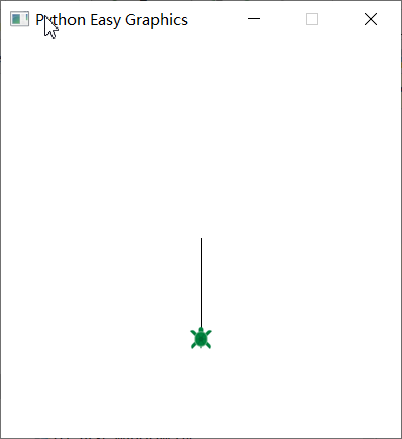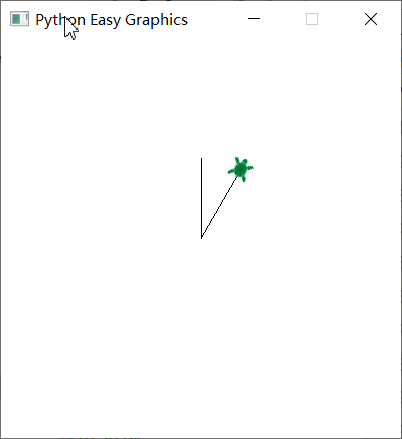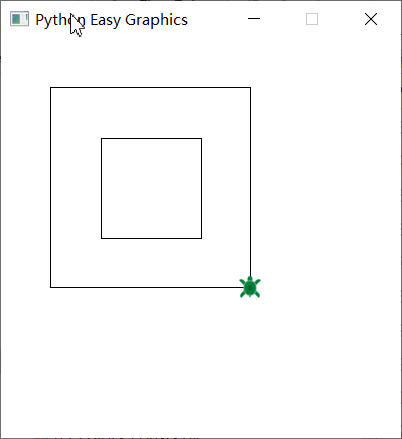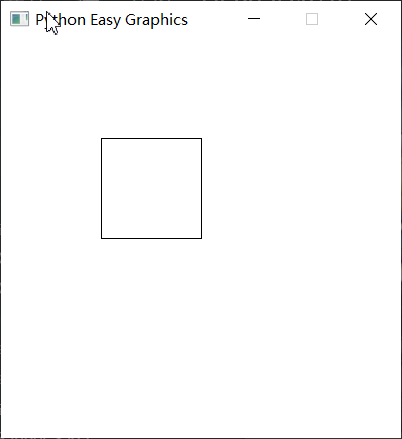# Basic Commands¶

In this tutorial, we will introduce basic commands of the turtle graphics.

## Move the turtle¶

forward(x) function move the turtle x steps forward. fd(x) is the short form of forward(x).

```from easygraphics.turtle import *

def main():
create_world(250,250)
fd(100)
pause()
close_world()

easy_run(main)
```backward(x) function move the turtle x steps backward. back(x) and bk(x) is the short form of backward(x).

```from easygraphics.turtle import *

def main():
create_world(400,400)
bk(100)
pause()
close_world()

easy_run(main)
```## Turning the turtle¶

right_turn(x) turns the turtle x degrees clockwise. right(x) and rt(x) are its short form.

left_turn(x) turns the turtle x degrees counter-clockwise. left(x) and lt(x) are its short form

The following program draws a 30 degree angle.

```from easygraphics.turtle import *

def main():
create_world(400,400)
fd(80)
bk(80)
rt(30)
fd(80)
pause()
close_world()

easy_run(main)
```## Speed of the turtle¶

We can use set_speed() to control the turtle’s moving speed. Speed is the more the fast, and 1 is the slowest. If you don’t need the animation, use set_immediate(True) to disable it.

## Pen Up and Pen Down¶

If you want to move the turtle without a trace, you can use pen_up and pen_down.

By default the turtle is in pen down state, which means its move will leave a trace.

If the turtle is in pen up state, its move will not leave a trace.

The following program use pen up and down to draw one square inside another.

```from easygraphics.turtle import *

def main():
create_world(400,400)

# draw the inside rectangle
for i in range(4):
fd(100)
lt(90)

# use pen_up to move the turtle without a trace
pen_up()
rt(135)
fd(70)
lt(135)
pen_down()

# draw the outside rectangle
for i in range(4):
fd(200)
lt(90)

pause()
close_world()

easy_run(main)
```## Show and Hide the Turtle¶

When the drawing is finished, we can hide() the turtle.

And show() makes the turtle visible again.

```from easygraphics.turtle import *

def main():
create_world(400,400)
for i in range(4):
fd(100)
lt(90)

hide()
pause()
close_world()

easy_run(main)
```